# Imperial Speed (Velocity)

The most common Imperial units used to measure speed are

• Feet per second (abbreviated as fps) and
• Miles per hour (abbreviated as mph)

Let's have a look at a few examples to get an idea of just how fast these are.

## Feet per Second

Something that travels at a speed of $1 \text{ fph}$ covers a distance of 1 foot in every second.

We usually cover a distance of around 13-16,000 feet every hour when we walk. If you walk at 1 foot per second, you are walking much more slowly than that as you will only cover 3,600 feet in one hour.

Think of $1 \text{ fps}$ as an extremely slow walking pace. Someone very elderly or unwell would probably walk more quickly than this. It's about the speed of a giant Galapagos tortoise.Light travels at a speed of $983,571,056 \text{ fps}$ in a vacuum. According to the theory of relativity, it's impossible to travel any faster than that.

## Miles Per Hour

If something is travelling at $1 \text{ mph}$, it covers a distance of 1 mile in every hour. This is a very slow speed.

We often use miles per hour to report the speeds of cars and other vehicles.

Let's have a look at a few examples of speeds in miles per hour.Our friendly garden snail can only manage a top speed of $0.06 \text{ mph}$.

$12.5 \text{ mph}$ is an easy cycling speed.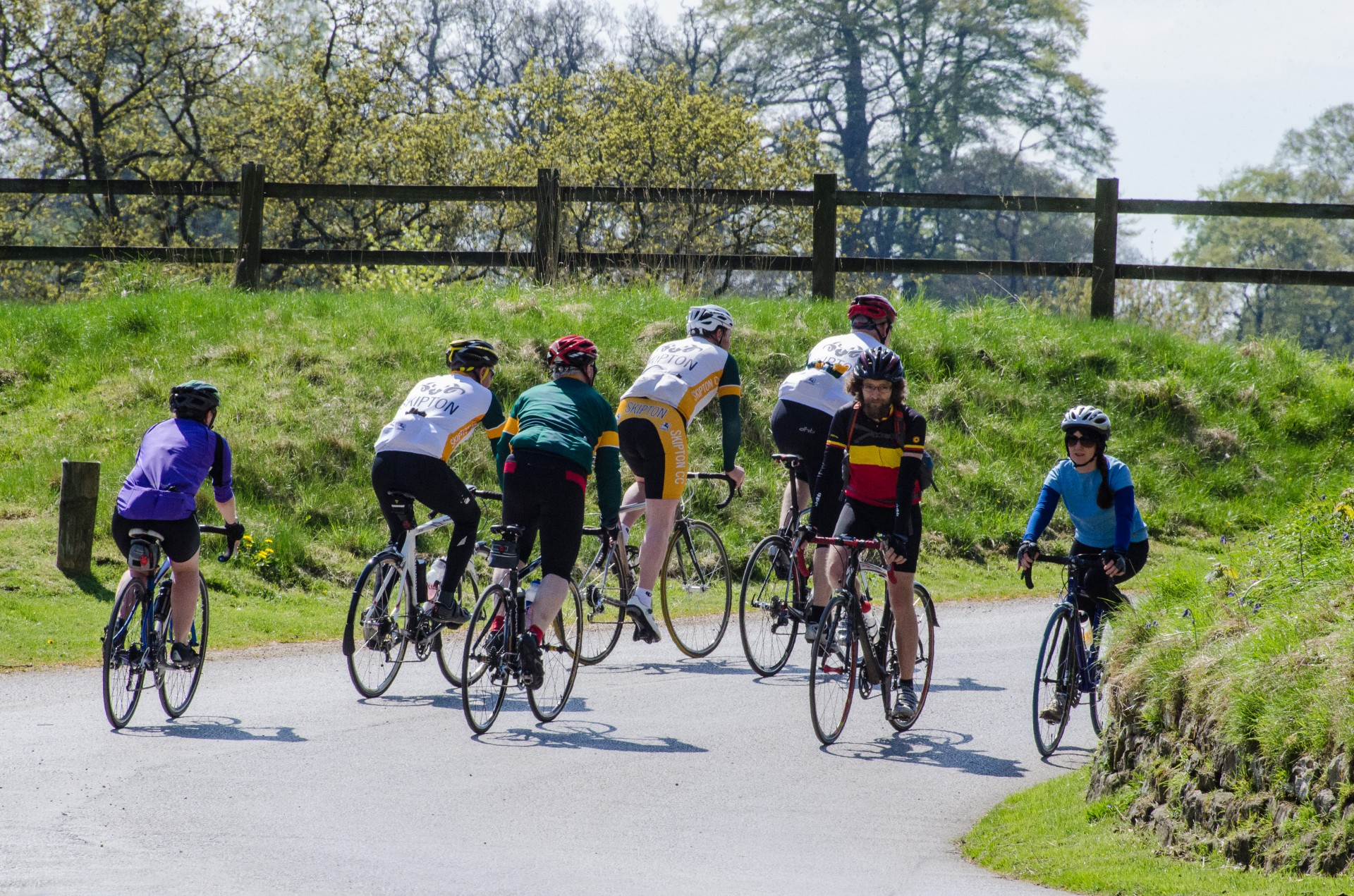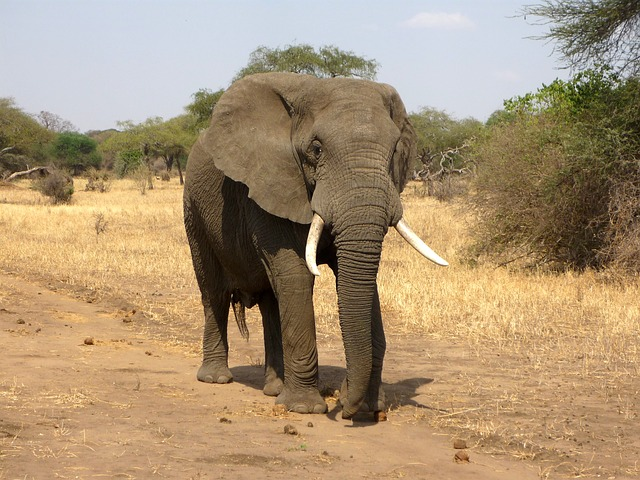$25 \text{ mph}$ is the speed limit (the maximum speed cars can legally travel at) for school zones. It is also the top speed of an African bush elephant.

$35 \text{ mph}$ is the maximum speed that cars can travel at in built up areas in many states. It is also the top speed of a large red kangaroo. It is possible to travel at this speed on a bicycle, but you would be going quite fast.A cheetah has a top speed of $74.6 \text{ mph}$, but the one in the picture is doing $0 \text{ mph}$. $55 \text{ mph}$ is the maximum speed at which cars can travel on highways in the United States. It is also about the speed of a goods train.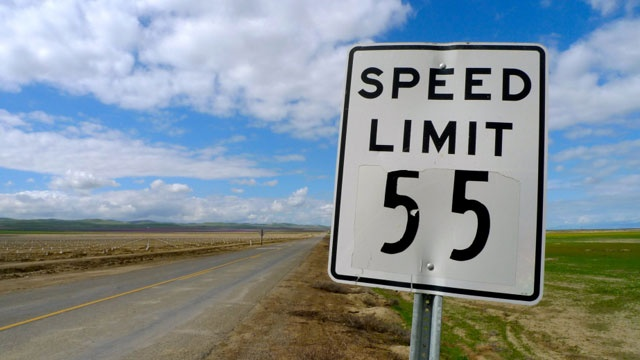The Japanese bullet train (shinkansen) travels at speeds of up to $200 \text{ mph}$ when it is in service. It has reached much higher speeds in test runs.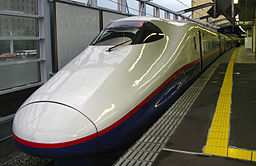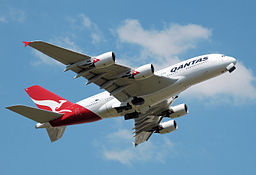The big Airbus A380 plane has a cruising speed of around $633.8 \text{ mph}$.

### Converting Speeds

It isn't too tough to convert speeds from $\text{ fps}$ to $\text{ mph}$. We have the following conversion factors (numbers to multiply by):

• $1 \text{ fps} = 0.6818 \text{ mph}$
• $1 \text{ mph}= 1.46666\dots \text{ fps}$

There are also conversion factors for converting speeds in Imperial units to speeds in metric units:

• $1 \text{ fps}$ is $0.3048 \text{ m/s}$
• $1 \text{ fps}$ is $1.09728 \text{ km/hr}$
• $1 \text{ mph}$ is $1.69034 \text{ km/hr}$

Let's do a couple of quick conversions as examples:

• A speed of $4 \text{ fps}$ is equal to a speed of $4 \times 0.3048 = 1.2192 \text{ m/s}$.
• A speed of $50 \text{ mph}$ is equal to a speed of $50 \times 1.69034 \dots = 18.52 \text{ km/h } (2 \text{ decimal places})$.

### What's the Difference Between Speed and Velocity?

A velocity is a vector quantity, so it must have a direction as well as a size. So, you can think of velocity as speed that is pointed in some direction.

For example, if someone tells you that they have been cycling at $15 \text{ mph}$ towards the north, then they are giving you their velocity. If they say that they have been cycling at $15 \text{ mph}$, then they are giving you their speed.

If someone takes a round trip from their home to the shops and then back again, their average velocity for the trip will be $0 \text{ mph}$ because they have ended up back where they started from. However, their average speed for the trip is likely to have been much higher. Otherwise, they couldn't have gone anywhere at all!

### Description

• Metric numbers
• Speed
• Conversions

and several other topics

### Audience

Year 10 or higher, some chapters are suitable for Year 8+ students

### Learning Objectives

Learn about measurement and related topics

Author: Subject Coach
You must be logged in as Student to ask a Question.